# Free Vibration of a Spring Mass System

1.5 Differential Equation for a spring-mass system

Let us consider a spring-mass system as shown in Fig. 1.1. The system is constrained to move in the vertical direction only along the axis of the spring. Let k and m be the stiffness of the spring and the mass of the block, respectively.

Let x be the position of the mass at any instant from the equilibrium position of the mass and it is assumed that x is positive in the downward direction and negative in the upward direction. In the spring-mass system only one coordinate is enough to describe the position of the mass at any time, and hence, it is single degree-of-freedom system. Here the coordinate is x .

###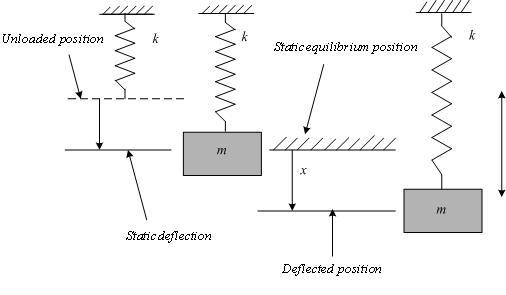Fig.1.1: Spring-mass system

###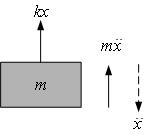Fig. 1.2: Free Body diagram of the mass

The free-body diagram of the mass is shown in Fig.2. Applying D’Alembert’s principle, the equation of motion of the mass can be obtained as,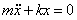(1.1)

The natural frequency of the system,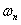is,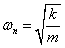(1.2)

Let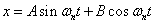(1.3)

be the solution for this differential equation (1.1).

Initial conditions: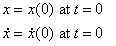(1.4)

Substitution of Eq.1.3 into Eq.1.1 and application of Eq.1.4 yields,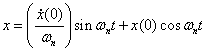(1.5)

Time period:

The time taken to complete one cycle, is,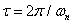(1.6)

It is important to note that, even though there is no specific damper attached to the system, there will always be the presence of damping of very small in amount. Because of this the response obtained from experiment always corresponds to a small amount of damping.

Let c be the damping coefficient, then the equation of motion changes to,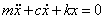(1.7)

Damping ratio ( ζ ):

It can be defined as the ration of the damping coefficient to the critical damping coefficient.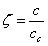(1.8)

where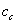is the Critical damping coefficient, given by: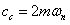(1.9)

If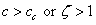, the system is said to be over-damped system.

If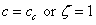, the system is said to be critically damped system.

If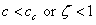, the system is said to be under-damped system

Following the same procedure as described above and by applying the initial conditions, the solution of the differential equation can be obtained as.

For Over-damped system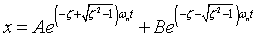(1.10)

Where,

###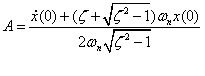and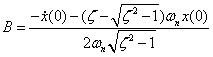For Critically-damped system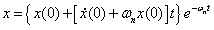(1.11)

For Under-damped system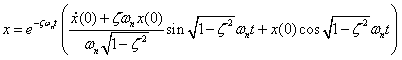(1.12)

The damped natural frequency of vibration is given by,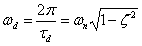(1.13)

### Where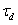is the time period of the oscillation:=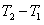The motion governed by this solution is of oscillatory type whose amplitude decreases in an exponential manner with the increase in time as shown in Fig. 3.

###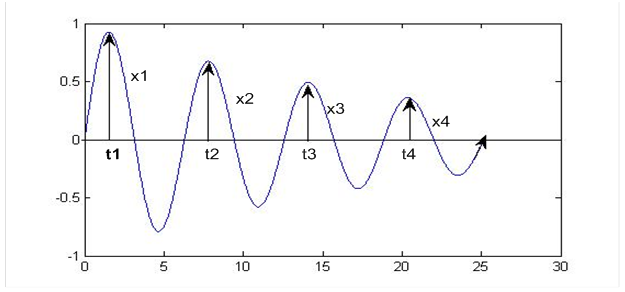Fig. 1.3: Displacement-time plot of an under-damped system with general initial conditions

The logarithmic decrement is given by,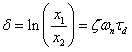(1.14)

Substituting Eq.1.13 into Eq.1.14,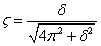(1.15)